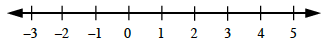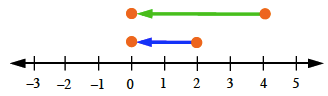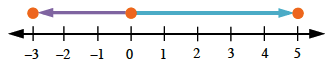### Home > CC1 > Chapter 3 > Lesson 3.2.4 > Problem3-128

3-128.

Evaluate each absolute value expression below. Homework Help ✎

Absolute value means distance away from zero on a number line.

Consult the number line below. How far is each value from 0?1. $| 2 | + | 4 |$2. $| - 3 | + | 5 |$|−3| = 3

|5| =5

3 + 5 = 8

3. $| - 7 | + | - 1 |$

4. $- | 6 |$

− 6